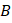# Consider the following statementandand identify the correct choice of the given answers (A) A zener diode is always connected in reverse bias (B) The potential barrier of ajunction lies between 0.1 to 0.3approximately a)andare correct b)andare wrong c)is correct butis wrong d)is wrong butis correct

## Question ID - 151903 :- Consider the following statementandand identify the correct choice of the given answers (A) A zener diode is always connected in reverse bias (B) The potential barrier of ajunction lies between 0.1 to 0.3approximately a)andare correct b)andare wrong c)is correct butis wrong d)is wrong butis correct

3537is correct butis wrong

Next Question :
 If 110 g of a salt is present in 550 g of solution then the concentration of solution will be (a) 50% (b) 40% (c) 20% (d) 10%